# Printable Fraction Decimal Percent ConversionJan 07, 2022By Printablee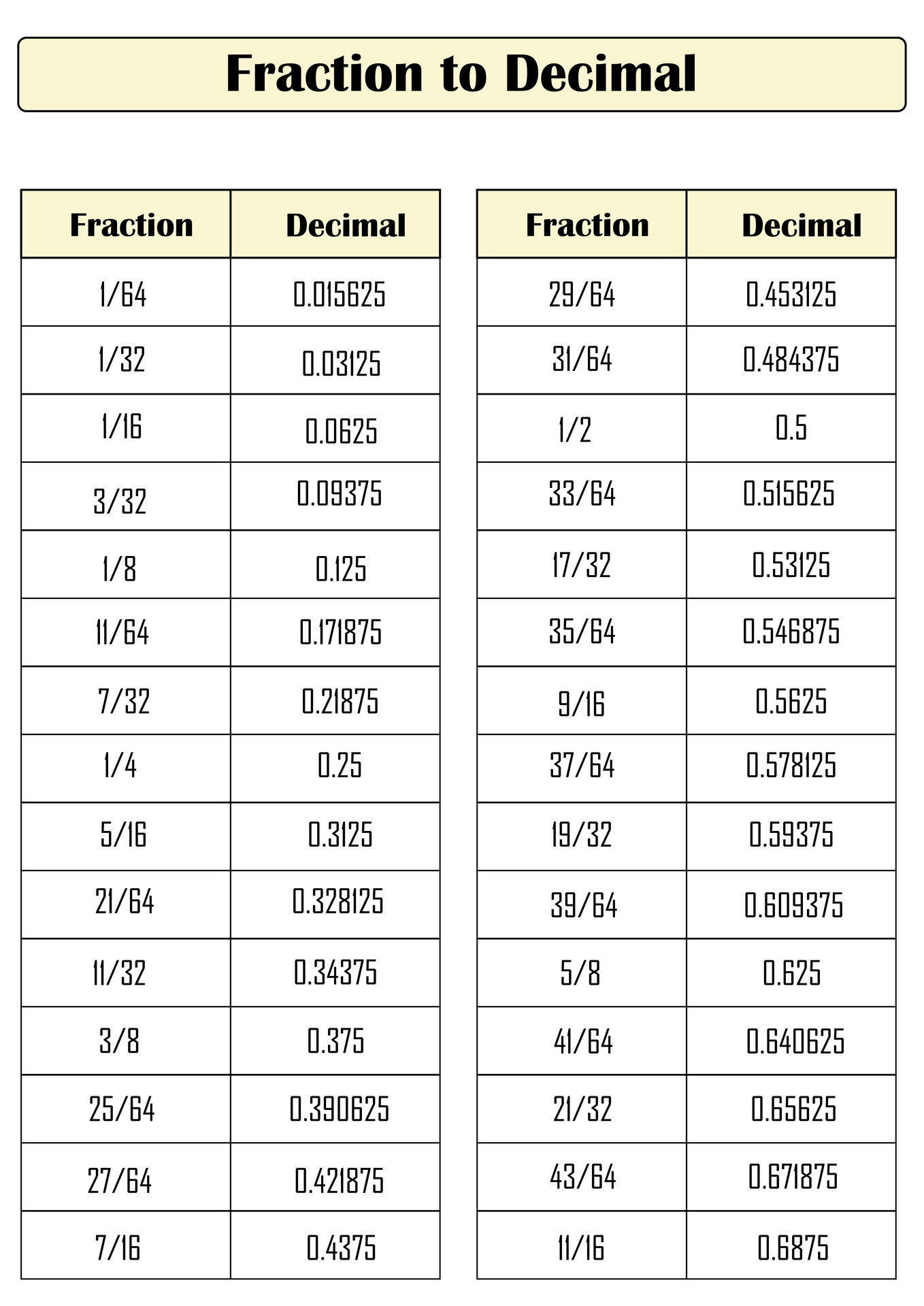### What is the connection between fractions, decimal and percent?

When you study Math, you will get lessons about conversion. Convert math to connect fractions, decimal and percent. What's interesting about this conversion is the way it is done differently, but the number values in the fractions, decimal and percent remain the same. This has become a varied form of expression in mathematics. Take it easy, the formula used to do the conversion can be done on all numbers.

### Why do we need to do the conversion for fraction, decimal and percent?

The purpose of conversion is to make it easier for you to do calculations in Math. If you are confronted with tasks that involve fraction, decimal and percent simultaneously, it will be easier for you to convert them to one of the forms first. After getting the answer, you can convert to the form in the problem. Both fraction, decimal and percent have their respective values and advantages. Some people are more comfortable solving problems in fraction formation, others prefer decimal and percent. You can choose which best suits you and the types of basic calculations you are working on.

### How to get a fraction decimal percent conversion chart?

Math will always have the same pattern because there is a formula created to make it easier to solve. But times are evolving to make things easier. In Math, fraction decimal percent conversion charts are made with this purpose. You can have it by searching for templates provided on the internet. There are various attractive versions and displays of every chart on the internet. This will be one of the points of interest for children. In some Math textbooks, you will also find the chart. Each chart has a different number but the pattern and formula used are always the same.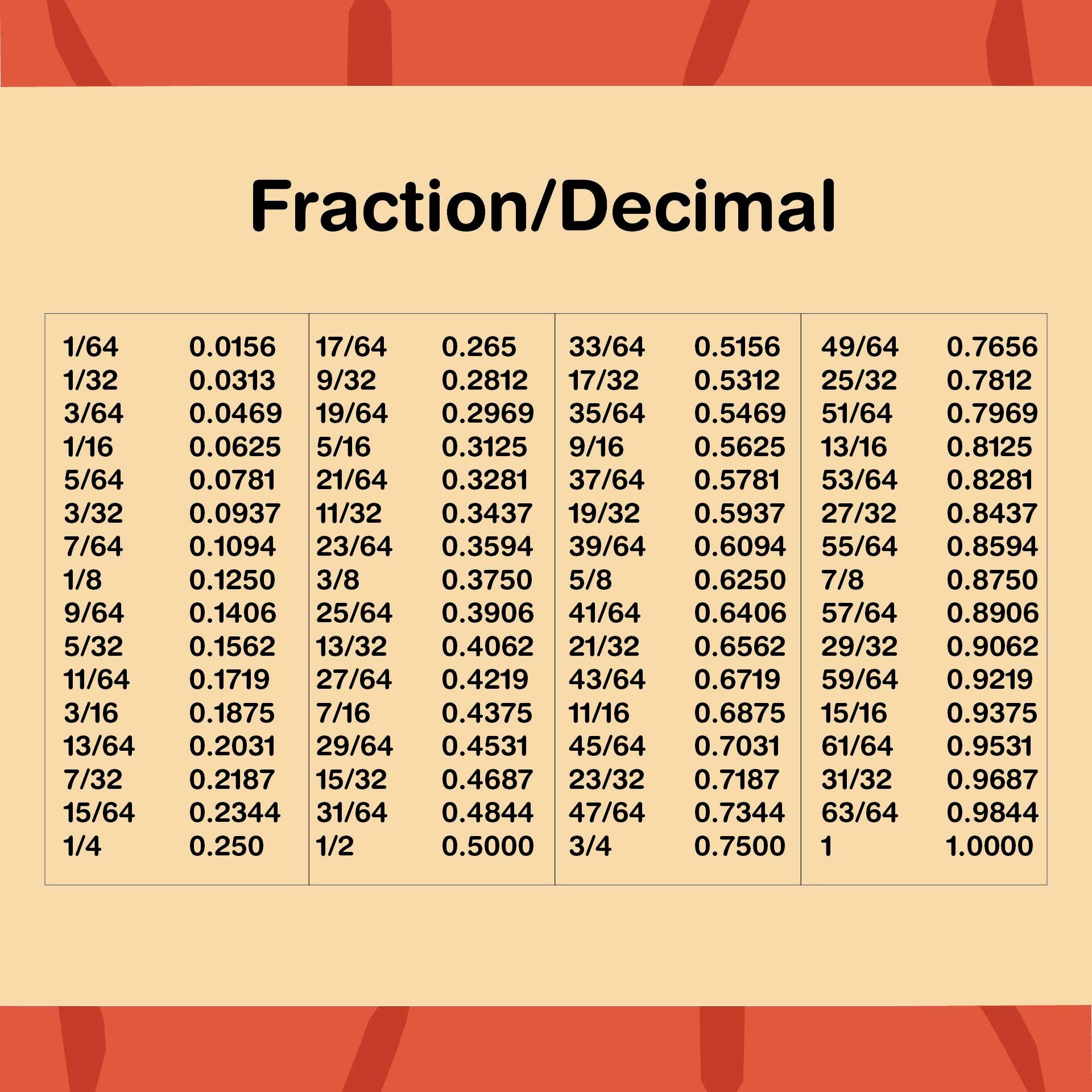We also have more printable other you may like:
Sign Printable First Day Of 6th Grade
Printable Directional Arrows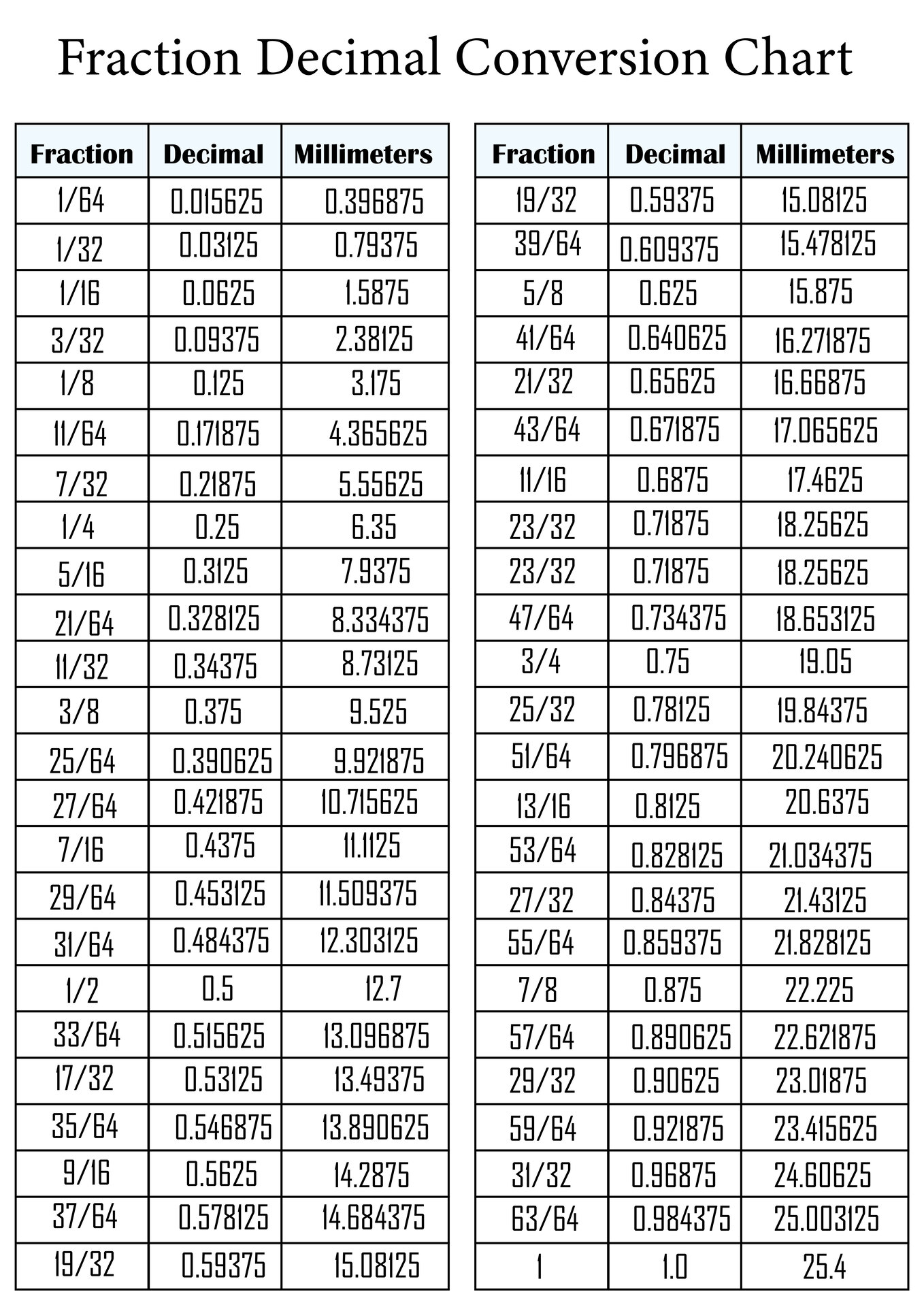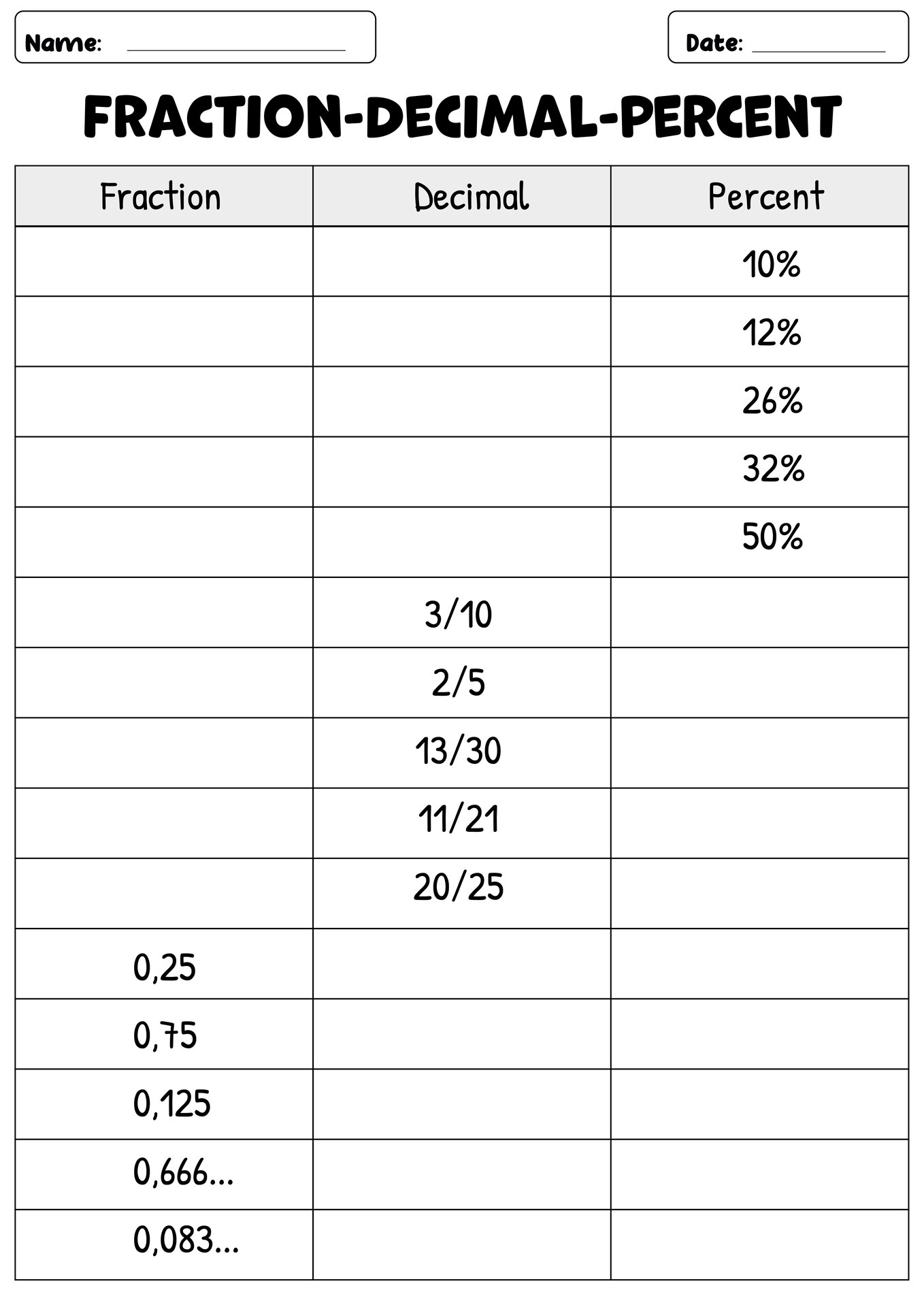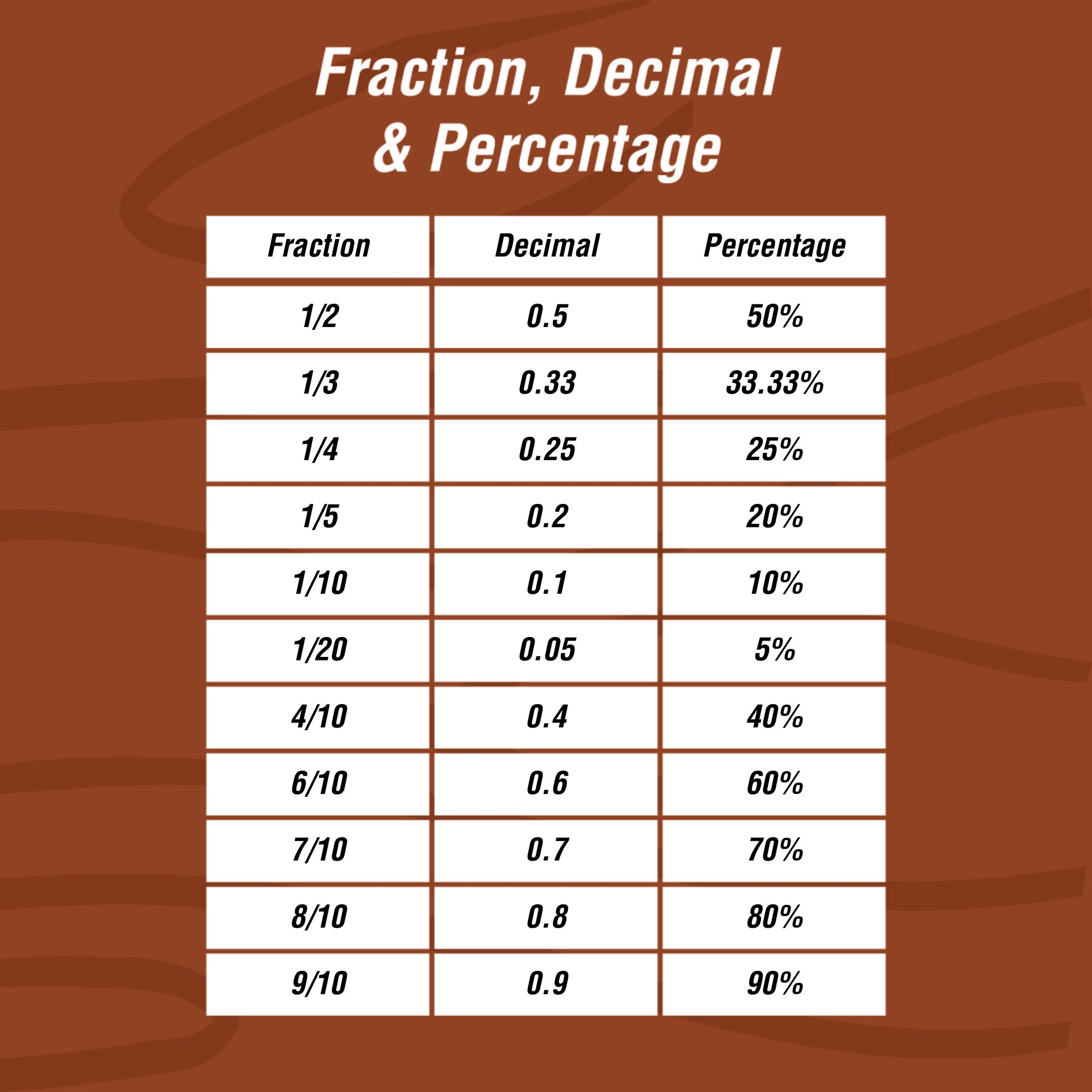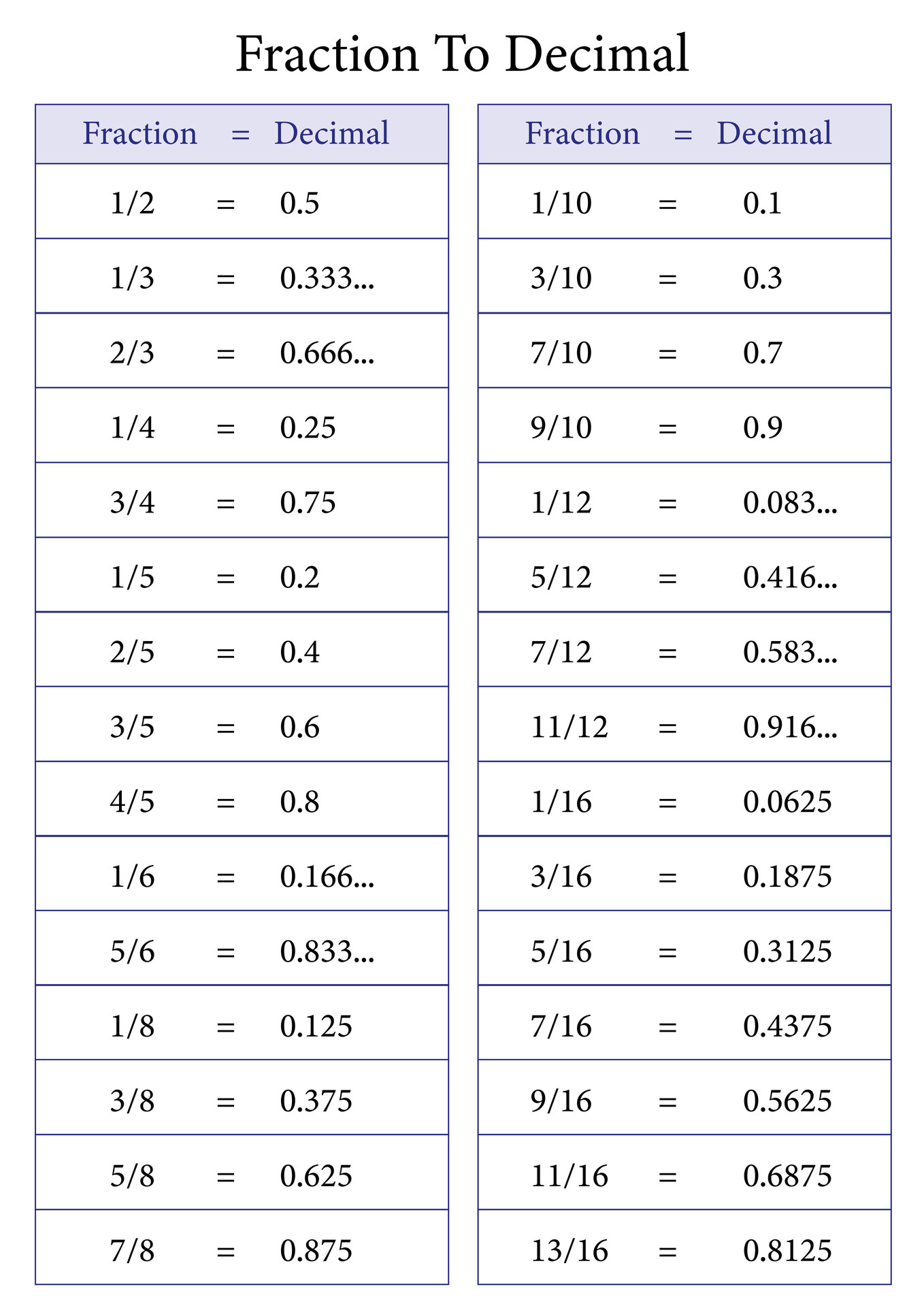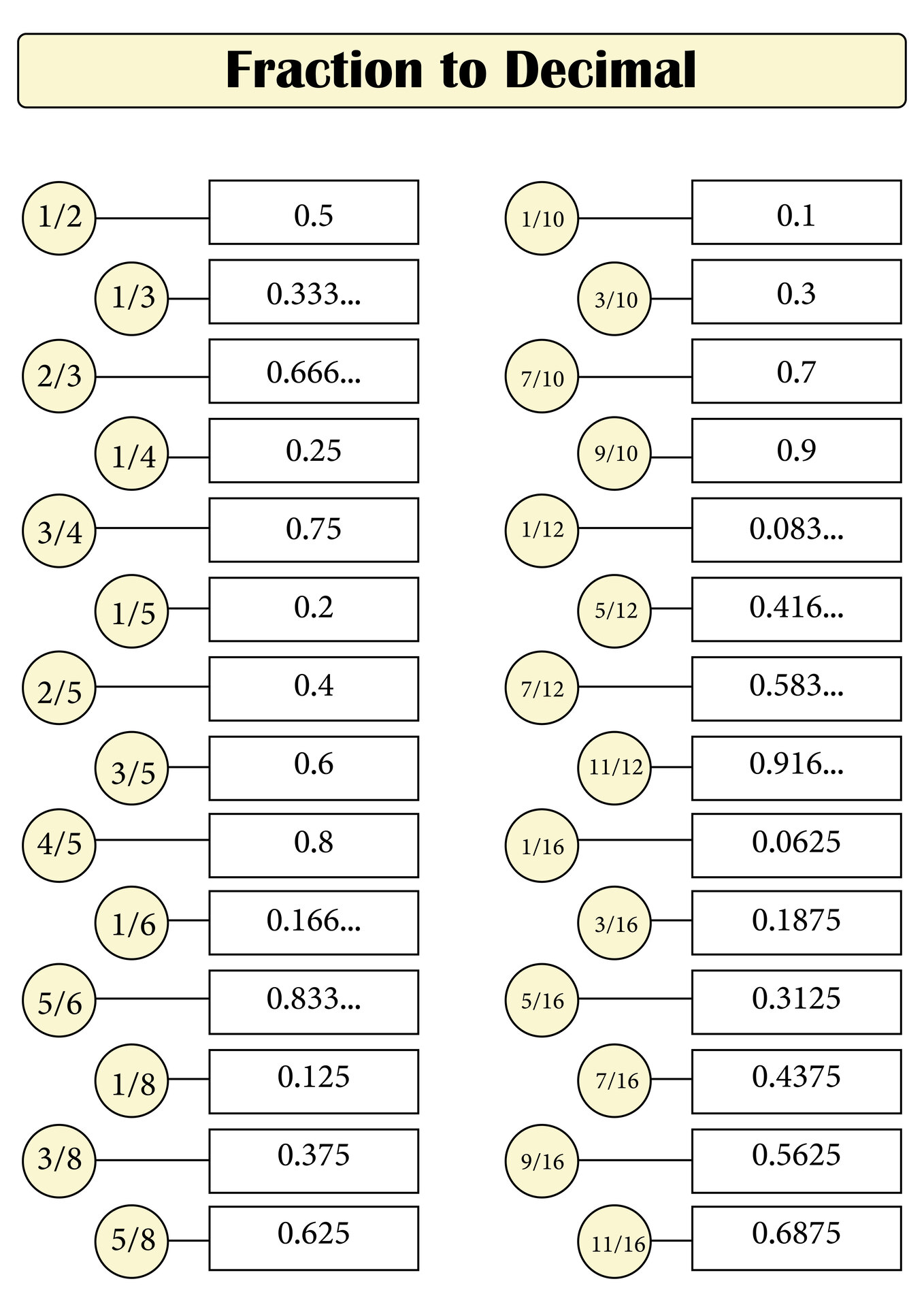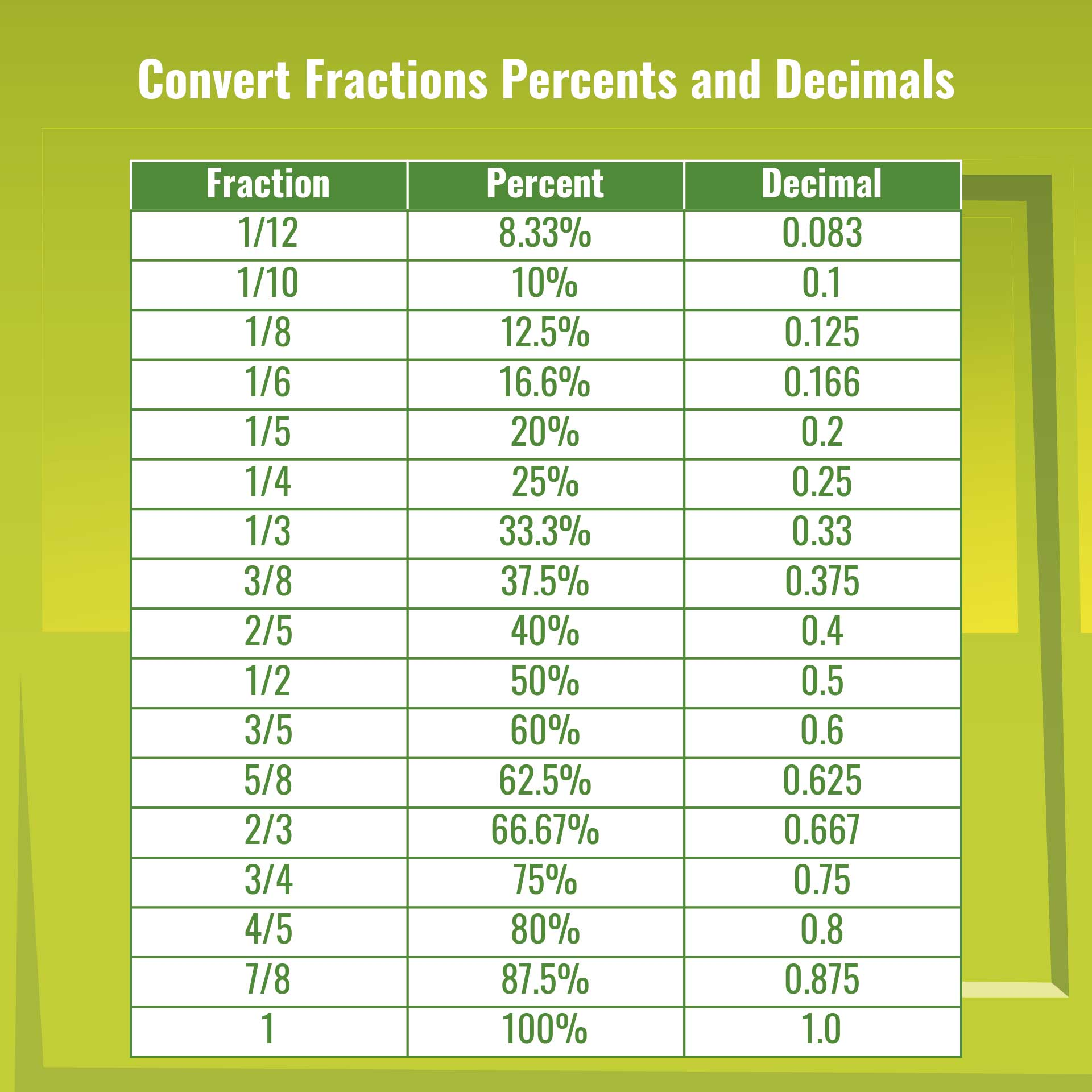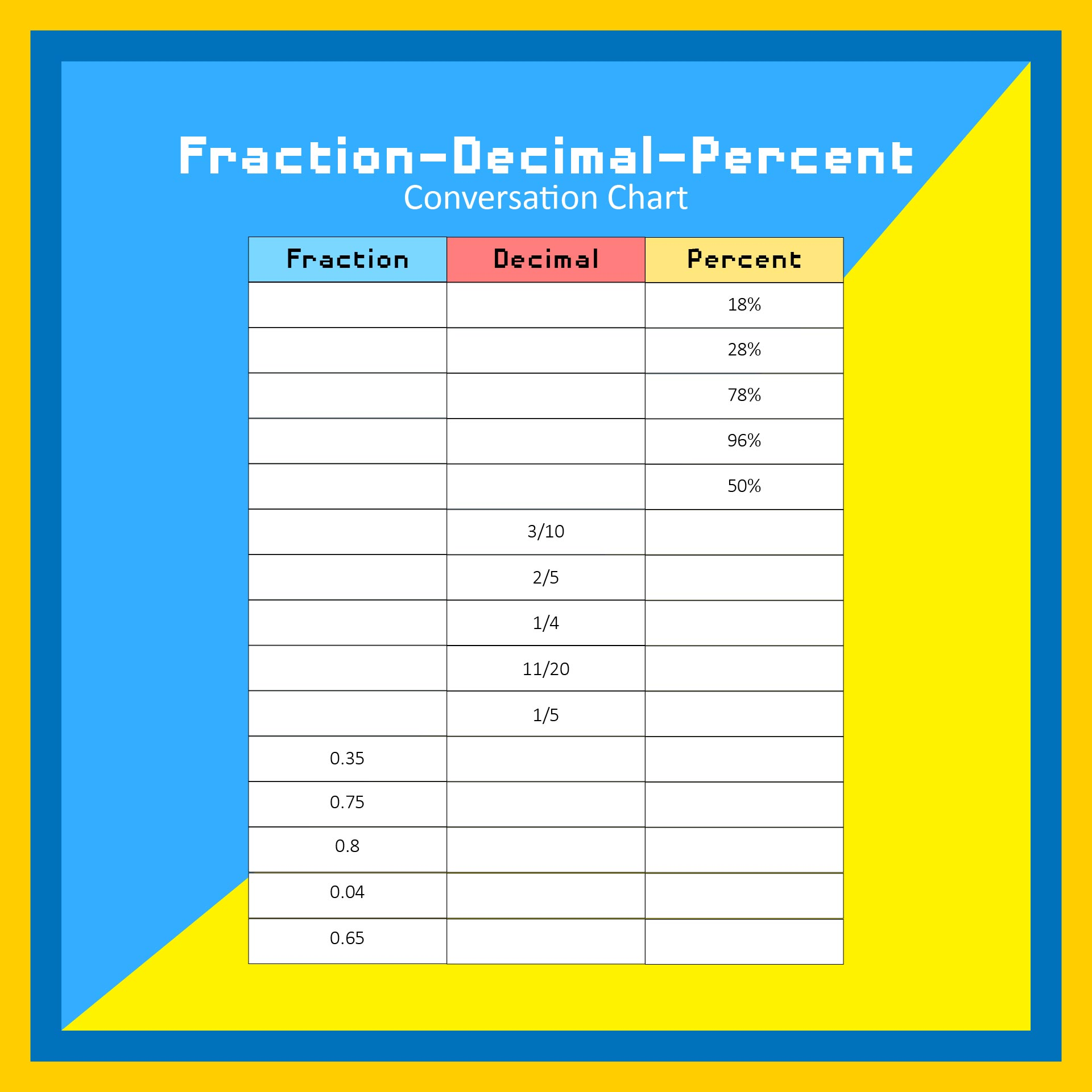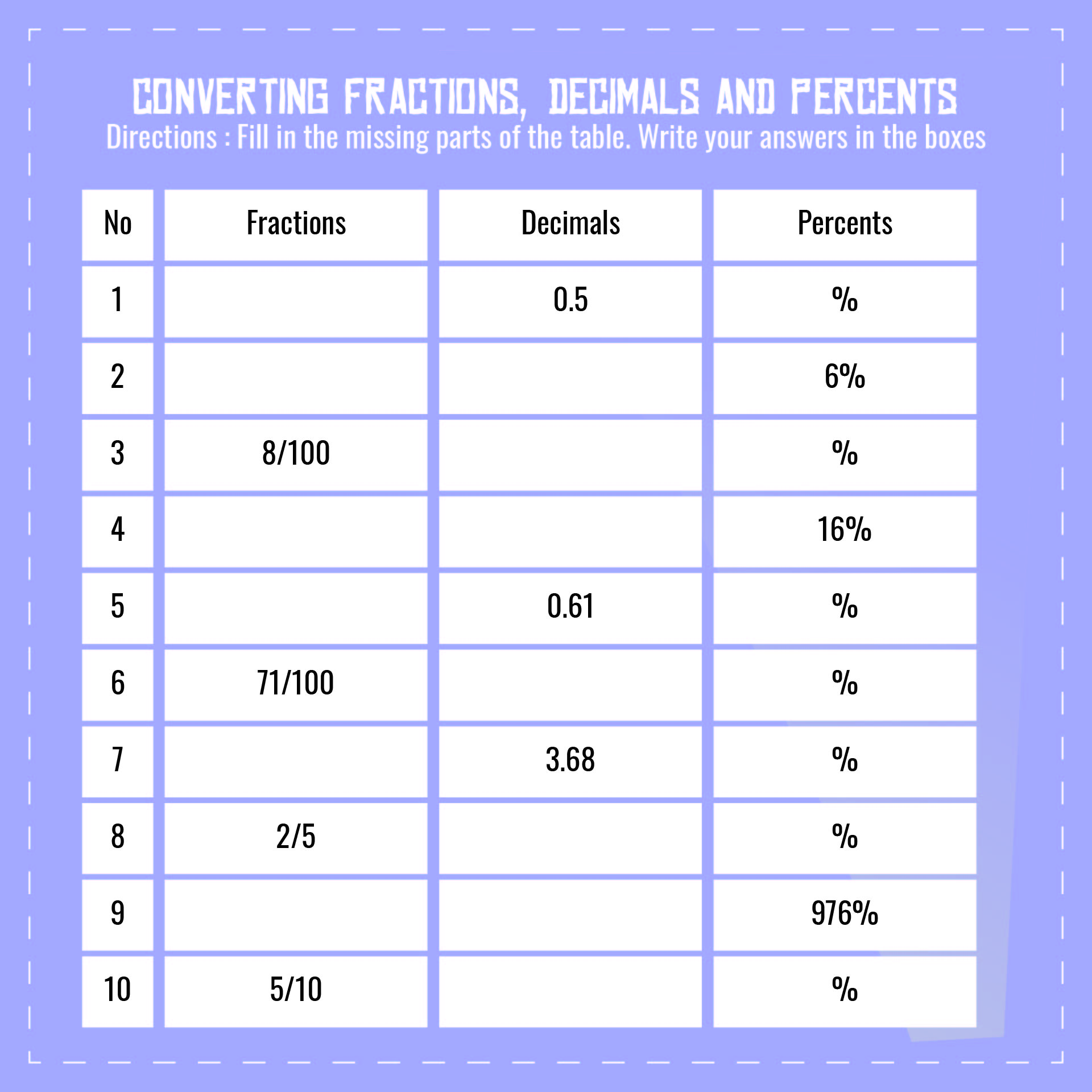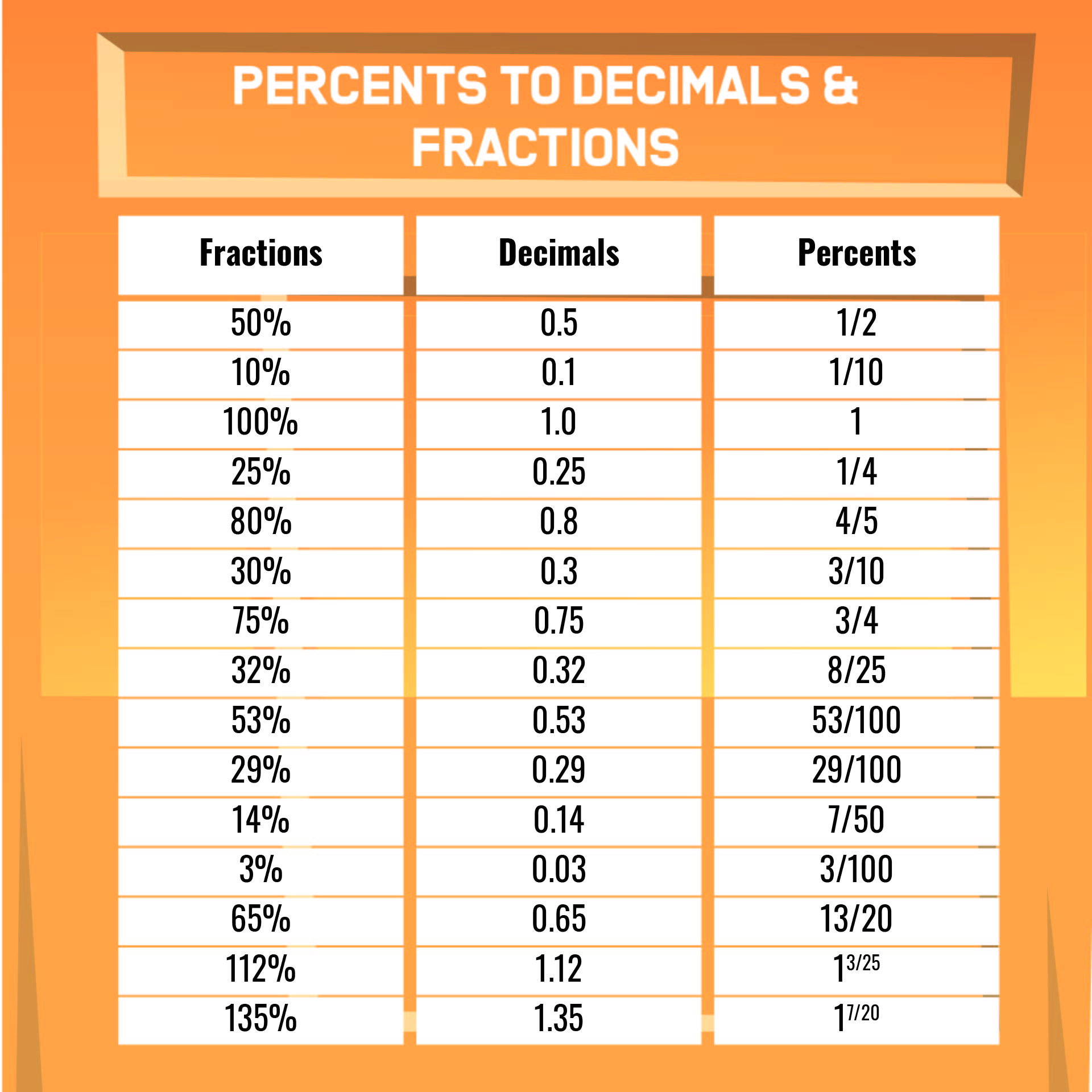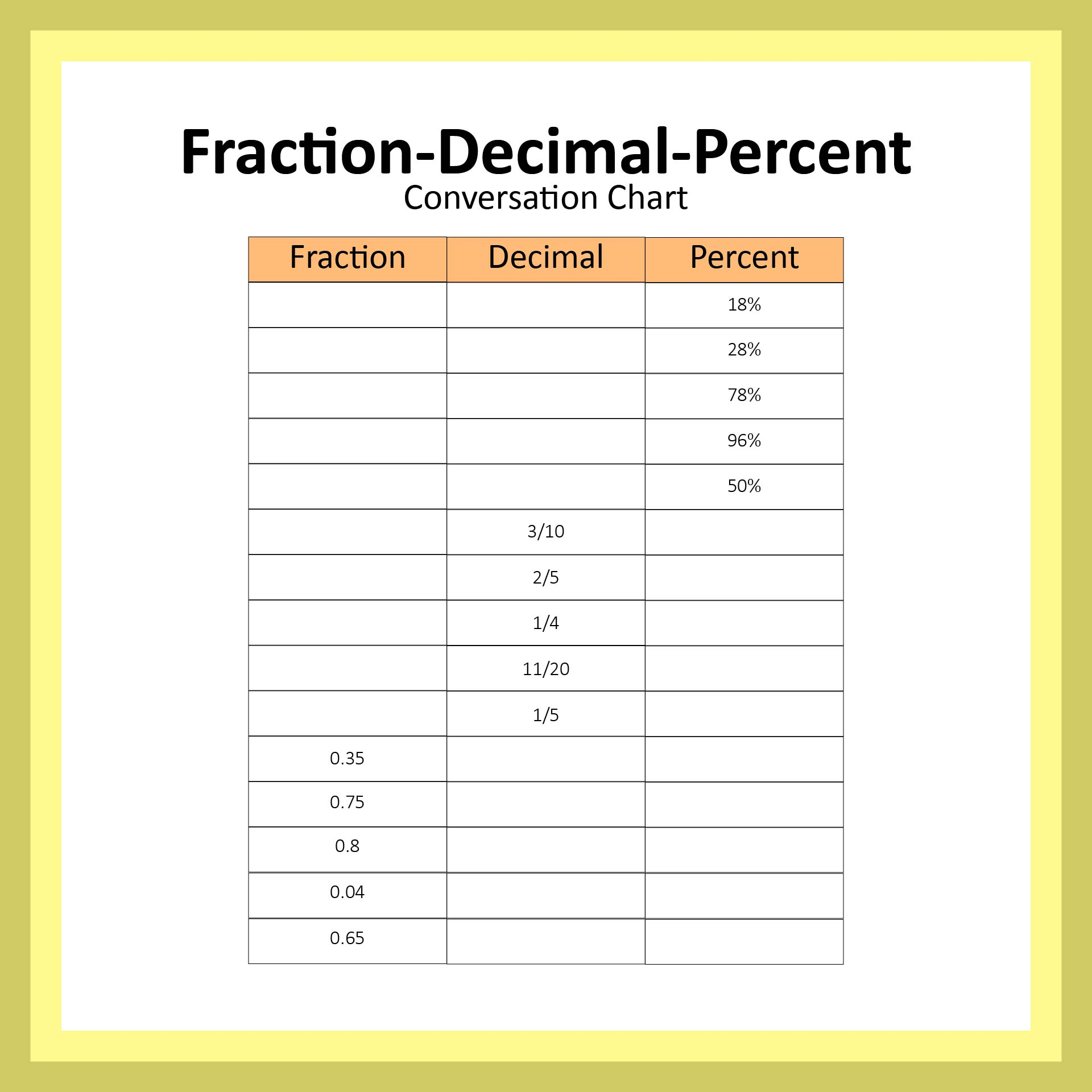### Is fraction decimal percent conversion chart overrated?

Using a chart for fraction decimal and percent conversion is sometimes too overrated for some people. Because what is done is conversion, it is better to only use notes in the form of formulas that can be applied to the conversion of the three forms. The numbers on the chart will make children more fixated on memorizing instead of understanding. Therefore, you can choose to use a template of fraction decimal percent conversion, a note, or your own reminder of fraction decimal and percent conversion. There are many ways to learn because every child has their own way of understanding and receiving learning.